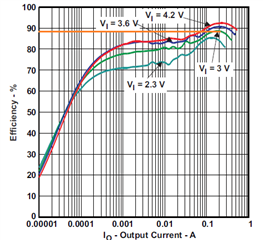If you have a related question, please click the "Ask a related question" button in the top right corner. The newly created question will be automatically linked to this question.

# TPS61240: Inductor Selection

Part Number: TPS61240

Hi,

I probably will use the TPS6140 (boost converter) to generate 5V 1W (0.2A) from a single cell Li-Ion battery (4.2V down to 3.0V).

I'm trying to compute the inductor value that I need. Here is my design procedure :1. Efficiency estimation : n=88%
2. Duty cycle : D=1-(VIN x n)/VOUT = 0.472
3. Average inductor current : ILavg=IOUT/(1-D)=0.379 A
4. Inductance value (dIL=0.2 x ILavg) : L=(VIN x D)/(dIL x fs)=5.34 uH

The problem is that the inductance is out of recommended value in the TSP6140 datasheet (1 to 2.2uH).

What should I do ?

P.S. Is there a tool for formatting equation in this forum ?

Best regards

• Hi

for such following integrated IC, please select the value suggested in the datasheet. refer to How to Select a Proper Inductor for Low Power Boost Converter